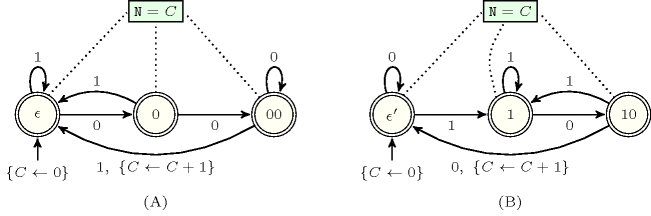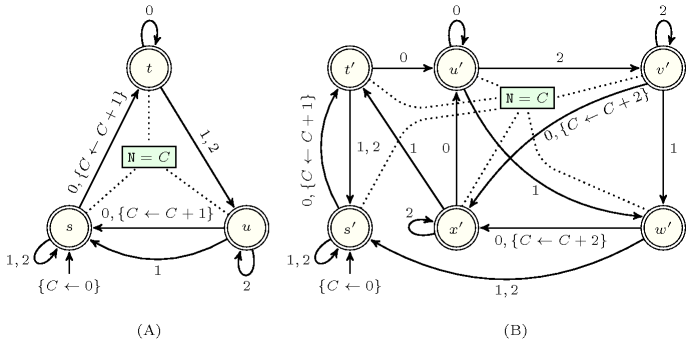### 3.7.214. Reverse of a constraint

A constraint which has a reverse constraint, where the reverse is defined in the following way. Consider two constraints $\mathrm{ctr}\left(\mathrm{𝑐𝑜𝑙},{r}_{1},\cdots ,{r}_{n}\right)$ and ${\mathrm{ctr}}^{\text{'}}\left(\mathrm{𝑐𝑜𝑙},{r}_{1},\cdots ,{r}_{n}\right)$ for which, in both cases, the argument $\mathrm{𝑐𝑜𝑙}$ is a collection of items that functionally determines all the other arguments ${r}_{1},\cdots ,{r}_{n}$.

The constraint ${\mathrm{ctr}}^{\text{'}}$ is the reverse constraint of constraint $\mathrm{ctr}$ if, for any collection of items $\mathrm{𝑐𝑜𝑙}$, we have the equivalence $\mathrm{ctr}\left(\mathrm{𝑐𝑜𝑙},{r}_{1},\cdots ,{r}_{n}\right)⇔{\mathrm{ctr}}^{\text{'}}\left({\mathrm{𝑐𝑜𝑙}}^{\mathrm{𝑟𝑒𝑣}},{r}_{1},\cdots ,{r}_{n}\right)$, where ${\mathrm{𝑐𝑜𝑙}}^{\mathrm{𝑟𝑒𝑣}}$ denotes the collection $\mathrm{𝑐𝑜𝑙}$ where the items of the collection are reversed. When constraints $\mathrm{ctr}$ and ${\mathrm{ctr}}^{\text{'}}$ are identical we say that constraint $\mathrm{ctr}$ is its own reverse.

The previous enumeration provides the list of reversible constraints where, for each reversible constraint, we give its reverse only when it is different from the original constraint.

Note that if a constraint can be represented by a counter deterministic automaton with one single counter that is only incremented and for which all states are accepting, then by computing the reverse automaton, the corresponding reverse constraint can be mechanically obtained. However note that the reverse automaton may be non-deterministic and may contain $ϵ$ transitions [Mohri2009]. Figure 3.7.57 gives an automaton counting the number of occurrences of words 001 in a sequence and its reverse automaton. Figure 3.7.58 provides an automaton with one counter and its reverse automaton that has a different number of states.

##### Figure 3.7.57. (A) Counter automaton returning the number of occurrences $𝙽$ of word 001 in a sequence, and (B) its reverse counter automaton (returning the number of occurrences $𝙽$ of word 100 in a sequence)##### Figure 3.7.58. (A) Counter automaton, and (B) its reverse counter automaton which has a different number of states